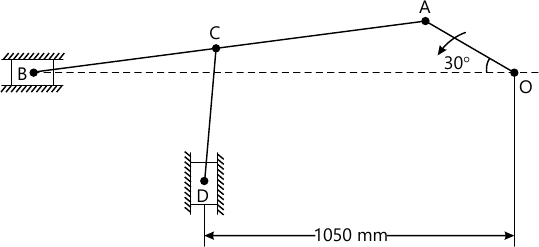MORE IN Theory of Machines - 1
MU Mechanical Engineering (Semester 4)
Theory of Machines - 1
December 2015
Total marks: --
Total time: --
INSTRUCTIONS
(1) Assume appropriate data and state your reasons
(2) Marks are given to the right of every question
(3) Draw neat diagrams wherever necessary

Attempt any four:
1 (a) What are the advantages and disadvantages of chain derive over belt derive?
5 M
1 (b) State and explain Work Energy principle.
5 M
1 (c) What do you mean by interference in involute gears?
5 M
1 (d) Classify followers in details.
5 M
1 (e) What are the different types of constrained motions?
5 M

2 (a) What do you mean by Coriolis component of acceleration? Draw all the direction of Coriolis component of acceleration.
6 M
2 (b) State and explain Law of Gearing.
6 M
2 (c) What is a Pantograph? Show that it can produce paths exactly similar to the ones traced out by a point on a link on an enlarged or reduced scale.
8 M

3 (a) In the mechanism as shown in the figure, the crank OA rotates at 40 rpm anticlockwise and gives motion on the sliding blocks B and D. The dimensions of the various links are OA=300 mm; AB=1200 mm; BC=CD=450 mm. For the given configuration determine i) velocities of sliding at B and D. ii) angular velocity of CD. Iii) Linear acceleration of D iv) angular acceleration of CD.14 M
3 (b) Sketch a polar velocity diagram of a HooKe's Joint and explain its salient features.
6 M

4 (a) A cam rotating at 150 rpm operates a reciprocating roller follower of radius 2.5 cm. The least radius of the cam is 5 cm and the stroke of the follower is 5 cm. Ascent and descent both takes place by uniform acceleration and retardation. Ascent takes place during 75° and descent during 90° of cam rotation. Dwell between ascent and descent is 60°. Sketch displacement, velocity and acceleration diagrams and mark salient features.
12 M
4 (b) In a crank and slotted lever quick return motion mechanism, the distance between the fixed centres is 240 mm and the length of the driving crank is 120 mm. Find the inclination of the slotted bar with the vertical in the extreme position and the time ratio of cutting stroke to the return stroke. If the length of the slotted bar is 450 mm, find the length of the stroke if the line of stroke passes through the extreme positions of the free end of the lever.
8 M

5 (a) State and explain D'Alembert's Principle.
4 M
5 (b) Two pulleys, one 450 mm diameter and the other 200 mm diameter are on parallel shafts 1.95m apart and are connected by cross belt. Find the length of the belt required and the angle of contact between the belt and each pulley. What power can be transmitted by the belt when the larger pulley rotates at 200 rpm, if the maximum permissible tension in the belt is 1×103 N and the coefficient of friction between the belt and the pulley is 0.25?
8 M
5 (c) Two mating gears have 20 and 40 involute teeth of module 10 mm and 20° pressure angle. If addendum on each wheel is such that path of contact is maximum and interference is just avoided, find the path of contact, are of contact and contact ratio. Also find the addendum for each wheel.
8 M

6 (a) The power is transmitted form a pulley 1 m diameter running at 200 rpm to pulley 2.25m diameter by means of a belt. Find the speed lost by the driven pulley as result of creep, if the stress on the tight and slack side of the belt is 1.4 MPs and 0.5 MPa respectively. The Young's Modulus for the material of the belt is 100 MPa.
4 M
6 (b) The reduction of speed from 360 rpm to 120 rpm is desired by the use of chain drive. The driving sprocket has 10 teeth. Find the number of teeth on the driven sprocket. If the pitch radius of the driven sprocket is 250 mm and the center to center distance between the two sprocket is 400 mm, find the pitch and the length of the chain.
8 M
6 (c) With the help of neat sketch derive the equation for the minimum number of teeth on a pinion for involute rack is order to avoid interference.
8 M

More question papers from Theory of Machines - 1